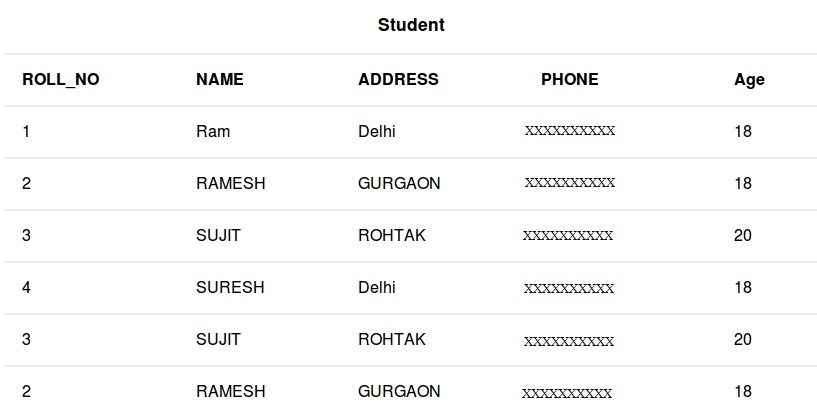# SQL | WHERE Clause

• Difficulty Level : Basic
• Last Updated : 19 May, 2022

WHERE keyword is used for fetching filtered data in a result set.

• It is used to fetch data according to a particular criteria.
• WHERE keyword can also be used to filter data by matching patterns.

Basic Syntax: SELECT column1,column2 FROM table_name WHERE column_name operator value;

```column1 , column2: fields int the table
table_name: name of table
column_name: name of field used for filtering the data
operator: operation to be considered for filtering
value: exact value or pattern to get related data in result ```

List of operators that can be used with where clause:Queries

• To fetch record of students with age equal to 20
`SELECT * FROM Student WHERE Age=20;`
• Output:
• To fetch Name and Address of students with ROLL_NO greater than 3
`SELECT ROLL_NO,NAME,ADDRESS FROM Student WHERE ROLL_NO > 3;`
• Output:

BETWEEN operator

It is used to fetch filtered data in a given range inclusive of two values. Basic Syntax: SELECT column1,column2 FROM table_name WHERE column_name BETWEEN value1 AND value2;

`BETWEEN: operator name `

value1 AND value2: exact value from value1 to value2 to get related data in result set.

Queries

• To fetch records of students where ROLL_NO is between 1 and 3 (inclusive)
`SELECT * FROM Student WHERE ROLL_NO BETWEEN 1 AND 3;`
• Output:
• To fetch NAME,ADDRESS of students where Age is between 20 and 30 (inclusive)
`SELECT NAME,ADDRESS FROM Student WHERE Age BETWEEN 20 AND 30;`
• Output:

LIKE operator

It is used to fetch filtered data by searching for a particular pattern in where clause. Basic Syntax: SELECT column1,column2 FROM table_name WHERE column_name LIKE pattern;

`LIKE: operator name `

pattern: exact value extracted from the pattern to get related data in result set. Note: The character(s) in pattern are case sensitive.

Queries

• To fetch records of students where NAME starts with letter S.
`SELECT * FROM Student WHERE NAME LIKE 'S%'; `
• The ‘%'(wildcard) signifies the later characters here which can be of any length and value.More about wildcards will be discussed in the later set. Output:
• To fetch records of students where NAME contains the pattern ‘AM’.
`SELECT * FROM Student WHERE NAME LIKE '%AM%';`
• Output:

IN operator

It is used to fetch filtered data same as fetched by ‘=’ operator just the difference is that here we can specify multiple values for which we can get the result set. Basic Syntax: SELECT column1,column2 FROM table_name WHERE column_name IN (value1,value2,..);

`IN: operator name `

value1,value2,..: exact value matching the values given and get related data in result set.

Queries

• To fetch NAME and ADDRESS of students where Age is 18 or 20.
`SELECT NAME,ADDRESS FROM Student WHERE Age IN (18,20);`
• Output:
• To fetch records of students where ROLL_NO is 1 or 4.
`SELECT * FROM Student WHERE ROLL_NO IN (1,4);`
• Output: